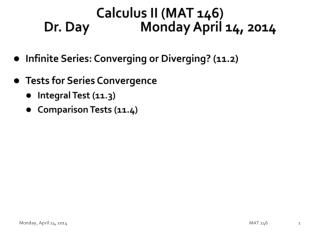DownloadDownload PresentationCalculus II (MAT 146) Dr. Day Monday April 14, 2014

# Calculus II (MAT 146) Dr. Day Monday April 14, 2014

Télécharger la présentation## Calculus II (MAT 146) Dr. Day Monday April 14, 2014

- - - - - - - - - - - - - - - - - - - - - - - - - - - E N D - - - - - - - - - - - - - - - - - - - - - - - - - - -
##### Presentation Transcript

1. Calculus II (MAT 146)Dr. Day Monday April 14, 2014 • Infinite Series: Converging or Diverging? (11.2) • Tests for Series Convergence • Integral Test (11.3) • Comparison Tests (11.4) MAT 146

2. Polynomial Approximators Our goal is to generate polynomial functions that can be used to approximate other functions near particular values of x. The polynomial we seek is of the following form: MAT 146

3. What is an Infinite Series? Our goal is to determine whether an infinite series converges or diverges. It must do one or the other. If the sequence of partial sums {si} has a finite limit as n −−> ∞, we say that the infinite series converges. Otherwise, it diverges. MAT 146

4. Notable Series The harmonic series is the sum of all possible unit fractions. MAT 146

5. Notable Series A geometric series is created from a sequence whose successive terms have a common ratio. When will a geometric series converge? MAT 146

6. Geometric Series (1) Create a geometric series to represent the repeating decimal fraction 0.45454545454545… and then determine the common fraction equivalent to this repeating decimal fraction. • (2) A geometric series has first term a1 = 1 and common ratio x, |x| < 1. • (a) Represent this using sigma notation and then show the first five terms of the series. • (b) Using your knowledge of convergent geometric series, determine a representation for this sum. MAT 146

7. Notable Series A telescoping sum can be compressed into just a few terms. MAT 146

8. Fact or Fiction? MAT 146

9. Series Convergence or Divergence We have defined sequences and series and have considered a few particular series—geometric series, harmonic series, telescoping sums—and explored the convergence/divergence of these series. Our first test related to this is • The Divergence Test also known as The nth-Term Test: If the sequence of terms that comprise an infinite series DO NOT have a limit of 0, then the series DOES NOT converge. The series diverges. We now turn our attention more fully to the fate of infinite series. What strategies do we have for determining whether an infinite series converges or diverges? MAT 146

10. A Series Convergence Test:The nth-Term Testalso calledThe Divergence Test MAT 146

11. MAT 146

12. MAT 146

13. MAT 146

14. MAT 146

15. MAT 146

16. MAT 146

17. MAT 146

18. MAT 146

19. MAT 146

20. MAT 146

21. MAT 146

22. MAT 146

23. Converge or Diverge? MAT 146

24. Converge or Diverge? (9) MAT 146

25. Converge or Diverge? (12) MAT 146

26. MAT 146

27. MAT 146

28. MAT 146

29. Assignments WebAssign • WA 11.2 due tonight! MAT 146